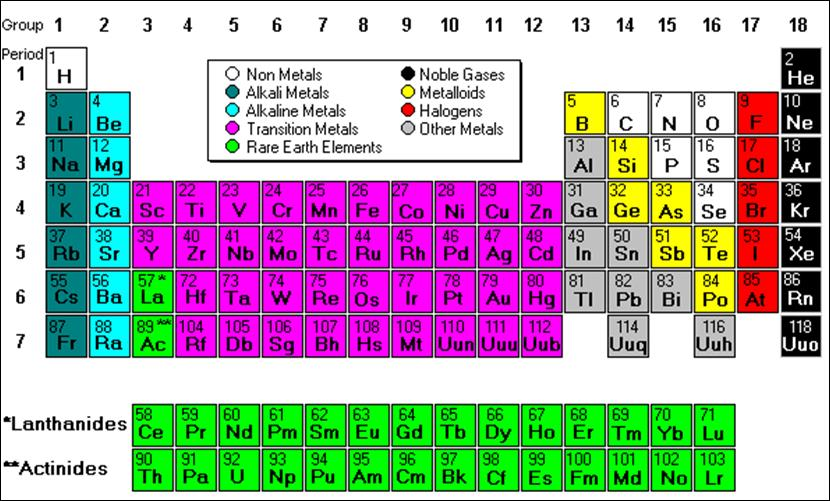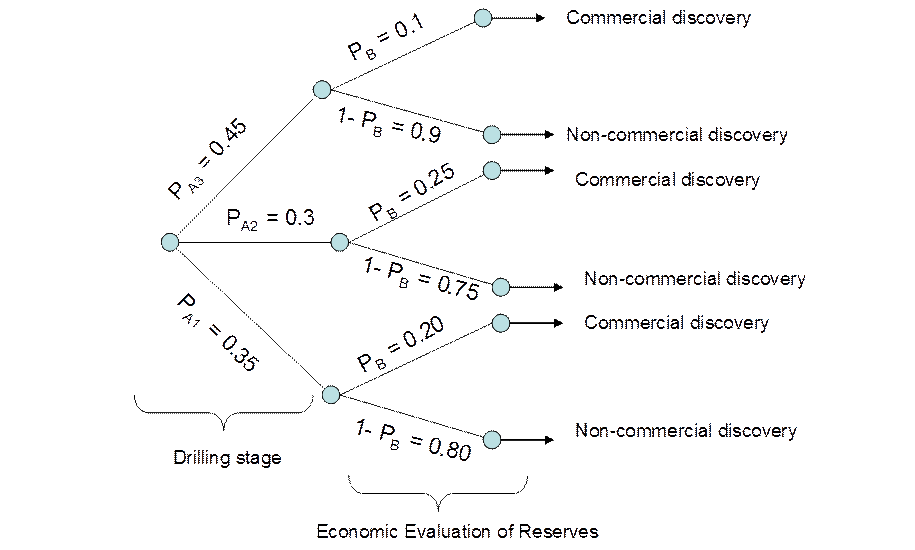Uncertainty is a predominant characteristic of the modern world. Quantifying uncertainty and making inferences from a bewildering array of data are increasingly valuable skills in the current world. Probability and Statistics are foundation tools needed for these skills and are prerequisites for our Mineral Economics Program. The following self test is designed to help you review the concepts that will be important to know for courses that deal with uncertainty or estimating statistical relationship.

Self Test 1 for Probability and Statistics

This test, constructed by Zauresh Atakhanova, Carol Dahl, and Arturo Vasquez has a mineral/energy slant and supplements the material in Schaum's Outline Theory and Problems of Probability and Statistics by Murray R. Spiegel. If you need more review refer to Schaum's outline.

# Sets

Sets, which are collections of objects, are at the foundation of Probability and Statistics. The 38 elements in groups 3 through 12 of the periodic table below are called 'transition metals.' As with all metals, the transition elements are ductile and malleable and they conduct electricity and heat. Their valence electrons are present in more than one shell. This is why they often exhibit several common oxidation states. The atomic mass or weight of an element is its average number of protons plus neutrons and the atomic number, listed in the left upper corner in the periodic chart below, is its number of protons (contributed by Carol Dahl).1. True False. We can describe the set 'T' for the transition metals by the property method using atomic numbers 'x'. So, we have T = { x: (21≤ x ≤ 30) or (39≤ x ≤48) or (72≤x ≤80) or (104≤x ≤112)}.

2. . Let the set T above, which is the transitional metals collection, be the universal set. Let A = {Gold (Au), Silver (Ag), Copper (Cu) } and B = {x | 26 < x < 30}. Where x again is the atomic number of the element. Then, A U B = {either A or B (or both)} = {Fe, Co, Ni, Cu, Zn, Au, Ag}

3. . Again let A = { Au, Ag, Cu} and B = { Fe, Co, Ni, Cu, Zn}. Then, A ∩ B = {elements in both A and B) = Cu}.

4. . Again let T, the transitional metals, be the universal set, A = {Au, Ag, Cu} and B = { Fe, Co, Ni, Cu, Zn}. Then, A ∩ B' = {All transition elements but Gold or Silver}

5. . Again let T, the transitional metals, be the universal set, A = {Au, Ag, Cu} and B = { Fe, Co, Ni, Cu, Zn}. A'UB' = {Anything but Copper}

6. . Again let T, the transitional metals, be the universal set, A = {Au, Ag, Cu} and B = { Fe, Co, Ni, Cu, Zn}. Then, B - A = {Fe, Co, Ni, Zn}.

7. . Again let T, the transitional metals, be the universal set, A = {Au, Ag, Cu} and B = { Fe, Co, Ni, Cu, Zn}. Then, A' - B' = {any element with atomic number between 26 and 30}

8. . Again let T, the transitional metals, be the universal set, A = {Au, Ag, Cu} and B = { Fe, Co, Ni, Cu, Zn}. Then (A ∩ B) U (A ∩ B') = {A}.

# Random Experiments, Sample Spaces and Events

9. Random variables are sets with probabilities attached to them. Let A be a set containing five metal samples of a certain polymetallic mine: A = {18k Gold (Au1), 14k Gold (Au2), Silver (Ag), Copper (Cu), Aluminum (Al)}. Suppose you draw a metal at random from set A with replacement. Each sample is equally likely to be drawn. The probability that you draw gold on the first draw is 2/5.

10. True False. In the last exercise, we worked with set A containing five metal samples. Suppose that 14k Gold sample was contaminated and you threw it away. So, your set is now A - Au2 = {Au1, Ag, Cu, Al}. You draw one sample with replacement at random to assay. The probability that you draw Gold or Silver is 1/4.

11. True False. A Petroleum Engineer is analyzing a set of oil samples from different new oil fields in the Middle East in order of study their properties for commercial purposes. She received 15 samples: S = {crude oil with 'x' equal distinct integer values of API degrees | 20o < x ≤ 35o). In the roster method S = {21, 22, 23, 24, 25, 26, 27, 28, 29, 30, 31, 32, 33, 34, 35}. In this set, heavy oils are considered to have an API degree between 21 and 24 and light oils to have an API degree between 31 and 35. The engineer has to show a report to the production manager of an oil company tomorrow morning. So, she needs to select a random sample without replacement of 3 crude oils so as to prepare this report as soon as possible. The probability that engineer selects light crude oils in the three draws is about 2.20% (contributed by Arturo Vasquez).

12. True False. In exercise 11, the probability that the engineer draws heavy oil in the first and second draws is (4/15)*(4/15).

13. True False. The company 'Quick Oil' has recently discovered two gas reservoirs with a high content of natural gas liquids. The reservoir A contains: A = {propane, butane, ethane, water}. The reservoir B probably contains: B = {heptane, hexane, octane, water}. The probability of producing Liquefied Petroleum Gas (LPG), which is propane and butane, from reservoir A is equal to 5%, whereas that the probability of producing LPG from reservoir B is equal to 90% (contributed by Arturo Vasquez).

# Calculation of Probabilities

14. A human resource company is looking for a mechanical engineer specialized in pipeline welding to help build a gas pipeline in Peru's jungle. In its database the company has a set of 52 engineers: 13 engineers from U.S.A, 13 from U.K, 13 from Russia and 13 from India. The years of experience for each country's professionals has a uniform distribution from 1 to 13 years with experience rounded to the closest year. Thus each country has an engineer with 1 year of experience, 2 years, . . . 13 years of experience. In a first step of the selection process, the company will select engineers at random. The probability of drawing an engineer with exactly ten years' experience or an engineer from Russia is 4/13.

15. In exercise 14, the probability of drawing neither an engineer with 4 years' experience nor an engineer from India is 10/13.

## Conditional Probability and Independent Events

16. A mineral engineer is working with ore samples to identity their quality. The engineer organizes the ores in a set by the predominant minerals in each ore: M = {gold1, gold2, gold3, silver1, silver2, silver3, zinc1, zinc2, zinc3, copper1, copper2, copper3, tin1, tin2, tin3, lead1, lead2, lead3}. The number '1' represents a high-quality mineral, the number '2' represents a middle-quality mineral, and 3 represents a low-quality mineral. The precious metals are gold and silver, whereas the other elements are non- precious metal. The engineer conducts two draws without replacement. The probability of selecting a low-quality gold ore in the first draw and of selecting a non-precious ore in the second draw is 1/17 ≈ 0.059 or 5.9% (Contributed by Arturo Vasquez).

17. In exercise 16, if the drawing process is conducted with replacement, the probability of selecting a silver ore in the first draw (event A), a zinc ore in the second draw (event 2), and a copper ore in the third draw (event 3) is 1/216.

# Total Probability and Bayes' Rule

18. A wildcat oil firm is exploring in a certain area of the Peruvian Amazon in order to confirm the existence of crude oil reserves. The wildcat is analyzing a statistical study about exploratory operations from another company which was looking for hydrocarbons in the same area years ago. A geologist of the wildcat found some probabilistic information in the form of a tree diagram. This information is about the probability of discovering oil in the area. There are three exploratory wells in the area: A1, A2, and A3. The probability of finding oil in these wells is shown in the tree diagram (drilling stage). However, there are certain probabilities that each well has commercial reserves. This information is determined by economic studies. The event of having commercial reserves 'after' discovering oil in a well is denominated 'B' in the tree diagram.The probability of having a commercial discovery of oil reserves in the area 21%. (Contributed by Arturo Vasquez).

19. True False. In exercise 18, we've calculated the probability of discovering commercial reserves. Because the probability of finding profitable reserves are not negligible for the exploration standards, the wildcatter initiates the exploration works. After drilling a lot in the area, the wildcat discovered commercial reserves. The probability that profitable reserves come from well A2 is 30%.

20. True False. This is an example of petroleum exploration using Bayesian analysis. In a certain area of Nigeria, which has a huge sedimentary deposit, seismic studies in the area found out the existence of two zones E1 and E2 which contain certain anomalies. Geologists have determined two probable states of nature: a) E1 could have 7 anomalies that contain no oil and 5 anomalies that contain oil, and b) E2 could have 9 anomalies that contain no oil and 3 anomalies that contain oil. They determines the following probabilities for those events: P(E1) = 0.33, whereas P(E2) = 0.67. A wildcatter drilled on one of the seismic anomalies and realized that the well is a dry hole, which we call event 'B'. This new information will help the wildcatter to revise original estimates of each of the hypothesized state of nature. The probability that the first anomaly drilled will be dry if in fact E1 is the true state of nature P(E1|B)=0.52. The probability that the first anomaly drilled will be dry if in fact E2 is the true state of nature P(E2/B) = 0.46 (contributed by Mansoor Al-Harthy and reviewed by Carol Dahl and Arturo Vasquez).

# Probability Using Combinatorial Analysis

21. True False. A mineral laboratory is analyzing 8 samples of copper, 3 samples of zinc, and 9 samples of aluminum. If 3 samples are drawn at random without replacement, the probability that 2 are copper and 1 is zinc equals 7/ 95.

22. True False. Out of 7 senior mining companies and 6 junior mining companies, a committee of 4 senior mining companies and 2 junior mining companies is to be formed. If any of the mining companies can be included, the number of possible committees is 525 (contributed by Claudio Valencia).

23. True False. A technical supervisor of an energy regulatory agency in certain developing country is checking the construction of an oil pipeline in the highlands. Specifically, he is looking for failures in the pipeline's welding. He can find 6 possible events after checking the X-ray shots of each pipeline junction: excellent welding (A), very good welding (B), good welding, (C) small porosities in the welding (D), large porosities in the welding (E), and a non-welded junction (F). If the probability of finding D is 1/6 and the probability of finding not D is 5/6 then the probability that event (D) occurs in 3 inspections of 5 if the events are mutually exclusive is 2.9% (contributed by Arturo Vasquez).